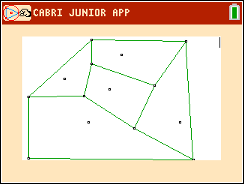Activities

•• Subject Area

• Math: Geometry: Quadrilaterals and Polygons

9-12

50 Minutes

• Device
•TI-84 Plus C Silver Edition
•TI-84 Plus CE

Voronoi DiagramsActivity Overview

In this TI-84 family activity, students will create Voronoi Diagrams and apply them to real world scenarios.

Objectives

• Students will create Voronoi Diagrams and apply them to real world situations.
• Students will draw triangles and find their circumcenters using perpendicular bisectors.
• Connect the use of Voronoi Diagrams with finding the Equations of lines in the form ax + by + c = 0 form, Where a, b, and c are all integers.

Vocabulary

• Voronoi Diagrams
• circumcenter
• seeds
•  Delaunay Triangulation
• perpendicular bisector